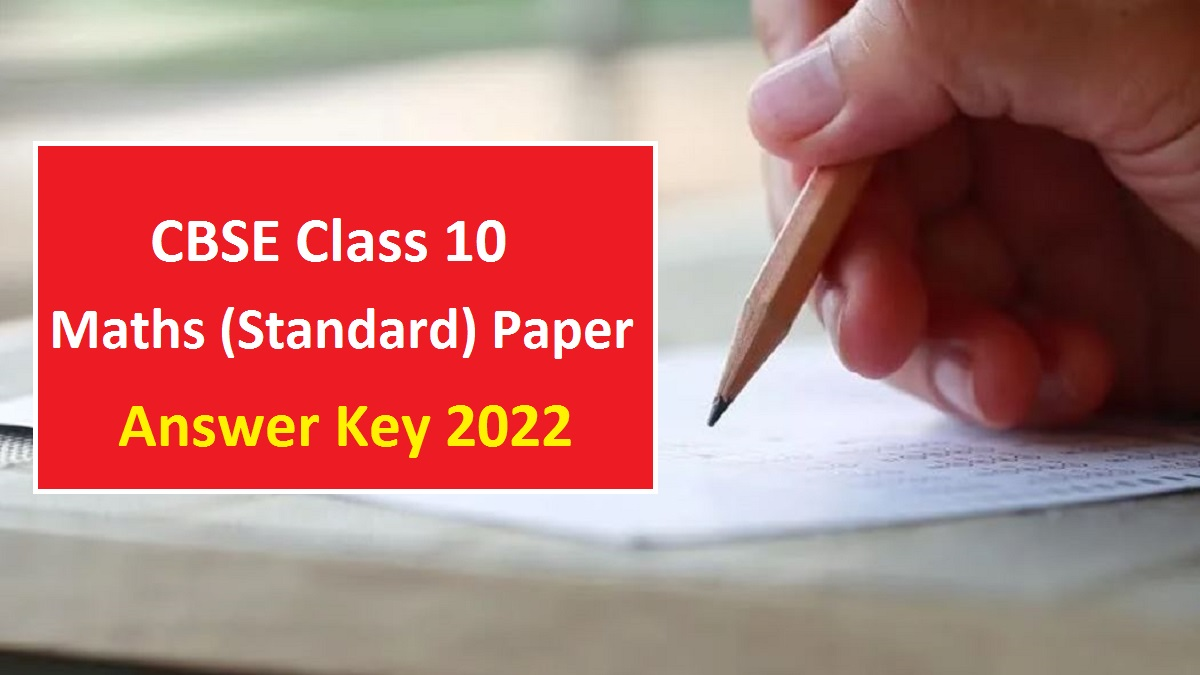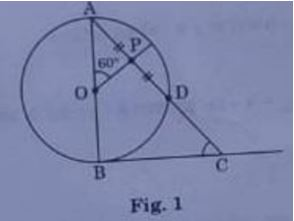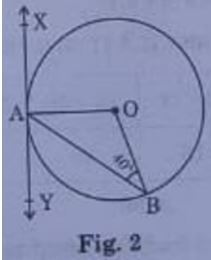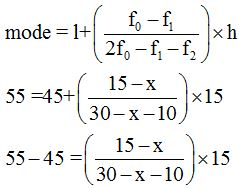# CBSE Class 10 Maths (Standard) Question Paper Solution (Term 2): Check Answers by Experts

0
72Check the solution of CBSE Class 10 Maths Standard Term 2 Question Paper 2022. All solutions are provided by the subject experts. Students can now cross check their answers and calculate the expected marks in their Maths paper.

CBSE Class 10 Maths Answer Key Term 2: CBSE Class 10 students must be waiting for the answer key of Maths paper that was conducted on 5th May, 2022. We have come here with the solutions created by the subject experts. Therefore, students can check now correct answers to all questions given in the CBSE Class 10 Maths (Standard) Term 2 Question Paper 2022. They can also download the question paper in PDF here and check the paper analysis along with the students’ feedback as well.

Check CBSE Class 10 Maths (Standard) Answer Key below:

1. In fig. 1, AB is diameter of a circle centered at O. BC is tangent to the circle at B. If OP bisects the chord AD and AOP = 60°, then find mC.Solution:

Given OP bisect the chord AD.

∠P = 900

and ∠B = 900

∠BOP = 1800 – 600 = 1200

Now, in quad. BOPC, applying angle sum property

∠P + ∠B + ∠O + ∠C = 3600

900 + 900 + 1200+ ∠C = 3600

∠C = 3600 – 300 = 600

OR

(b) In Fig. 2, XAY is a tangent to the circle centered at O. If ABO = 40°, then find mBAY and mAOB.Solution:

Given ∠ABO = 400

∠XAO = 900

OA = OB (Radii of same circle)

⇒∠OAB = ∠OBA

∴ ∠OAB = 400

Now, applying linear pair of angles property

∠BAY + ∠OAB + ∠XAO = 1800

∠BAY + 400 + 900 = 1800

∠BAY + 1300 = 1800

∠BAY = 500

Now, in ΔAOB,

∠AOB + ∠OAB + ∠OBA = 1800

∠AOB + 40+400 = 1800

∠AOB + 80= 1800

∠AOB = 1000

2. If the mode of the following frequency distribution is 55, then find the value of x.

 Class 0-15 15-30 30-45 45-60 60-75 75-90 Frequency 10 7 x 15 10 12

Solution:

Given that mode of frequency distribution is 55.

Here given mode =55

So, mode class is 45−60

lower limit (t) =45

class height (h) =15

then, f0 =15

f1 =x

f2=1010(30−x−10)=(15−x)15

10(20−x)=225−15x

200−10x=225−15k

15x−10x=225−200

5x=25

x=5

3. (a) In an AP if some of third and seventh terms is zero, find its 5th term.

Solution:

Let a and d be the first term and common difference of AP

nth term of AP, an​=a+(n−1)d

∴a3​=a+(3−1)d=a+2d

a7​=a+(7−1)d=a+6d

Given a3​+a7​=0

∴(a+2d)+(a+6d)=0

⇒2a+8d=0

⇒a+4d=0

Now, 5th term of given AP is,

a5=a+(5−1)d=a + 4d

a5= 0

OR

(b) Determine the AP whose third term is 5 and seventh term is 9.

Solution:

We know that

an​=a+(n−1)d

∴a3​=a+(3−1)d=a+2d

⇒ a+2d = 5    …..(i)

a7​=a+(7−1)d=a+6d

⇒ a+6d = 9      …..(ii)

Subtracting (ii) from (i), we have

4d = 4

d = 1

substituting d=2 in (i),

a + 2(1) = 5

a = 5-2

a=3

Thus, first term of required AP is

a = 3

second term = first term + common difference

a2 = 3+1 =4

Similarly, a3 = 4+1 =5, a4 = 6 and so on

Thus, the required AP is 3, 4, 5, 6, …….

4. Solve the quadratic equation x2 + 2√2x – 6 = 0.

5. Find the sum of first 20 terms of an AP whose nth term is given as an = 5 – 2n.

6. A solid piece of metal in the form of a cuboid of dimensions 11 cm x 7 cm x 7 cm is melted to form ‘n’ number of solid spheres of radii 7⁄2 cm each. Find the value of n.

Our experts are developing answers to the remaining questions. Keep visiting this page to know the correct answers to all questions asked in the CBSE Class 10  Term 2 Paper 2022.